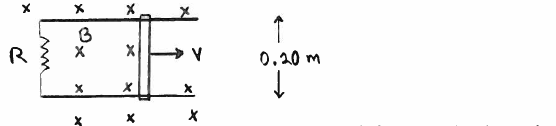# Solution: A conducting rod of length 0.20 m makes slides without friction on conducting metal rails, as shown in the sketch. The apparatus is in a uniform magnetic field that has magnitude B = 0.400 T and that is directed into the page. The resistance of the circuit is a constant R = 5.00 Ω.In a 3.00 s interval, how much work does the external force do on the bar if the bar is moving with constant velocity 12 m/s?

###### Problem

A conducting rod of length 0.20 m makes slides without friction on conducting metal rails, as shown in the sketch. The apparatus is in a uniform magnetic field that has magnitude B = 0.400 T and that is directed into the page. The resistance of the circuit is a constant R = 5.00 Ω.

In a 3.00 s interval, how much work does the external force do on the bar if the bar is moving with constant velocity 12 m/s?